# Multiple IF Statements in Excel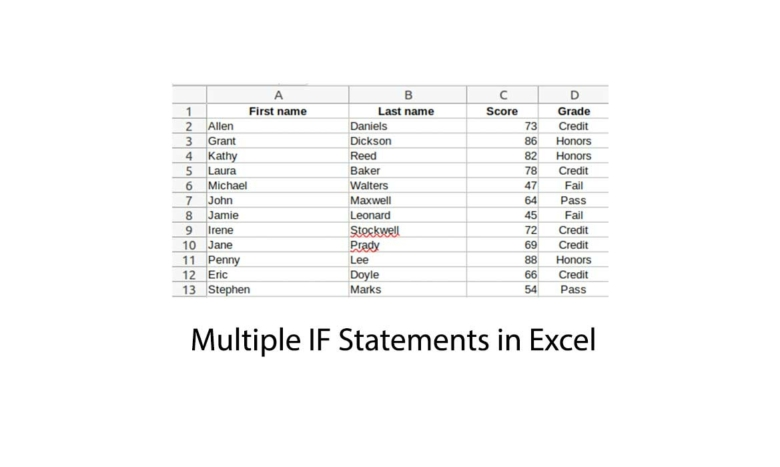We get this question quite a bit, “How do i run Multiple IF Statements in Excel?” – So today we’ll go over this in detail about first learning about why you would want to use multiple IF statements in excel or a worksheet.

IF is a conditional statement available in almost every programming language.

It evaluates an expression, which if true, executes any containing statements.

Successive if statements can independently test for a variety of conditions and perform the corresponding actions.

```IF (condition A is true) perform Action 1 IF (condition B is true) perform Action 2 IF (condition C is true) perform Action 3```

The above IF statements act independently of each other.

The outcome of condition A does not affect any of the following IF statements.

```IF (the hardware store is open) go inside and buy screws IF (the supermarket is open) go inside and buy groceries IF (the barber is open) get a haircut```

All of the above actions take place independently of each other.

Getting a haircut is not affected by whether the hardware store and supermarket are open or closed.

It is completely reliant only on whether the barber shop is open.

In most programming languages, IF can optionally be used with ELSE, so that if a condition is not met, then another course of action is followed.

```IF (door one is not locked) open it and go through ELSE try the second door.```

In this case, one of two actions is taken depending on the outcome of the test condition.

If the first door is not locked, go through, otherwise try door two.

## Running Multiple IF Statements

IF statements can also contain other IF statements, a practice known as nesting.

```IF (test score is 50 or above) IF (test score is 65 or above) IF (test score is 80 or above) passed with honors ELSE passed with credit ELSE passed ELSE failed```

To receive a pass with honors, the test score must be 50 or above so that it passes the first condition, it must then be 65 or above so that it passes the second condition, and finally, it must be 80 or above.

If the test score is 73, then it would fail at the third test condition (test score is 80 or above) and divert to the corresponding ELSE statement which gives a “passed with credit”.

In this scenario, each ensuing condition depends on all previous conditions having been met first.

IF statements are an important part of any programming language since they help direct the flow of the program.

They are the basic and most common form of decision making.

But Excel is not really a programming language, neither does it have an IF (or ELSE) statement.

It does however, have an IF function that can perform all of the above IF constructs, including multiple and nested IFs.

In fact, the Excel IF function is one of the most commonly used functions in Excel formulas.

The following sample sheet, with student names and their respective exam scores, will be used to show the IF function in action.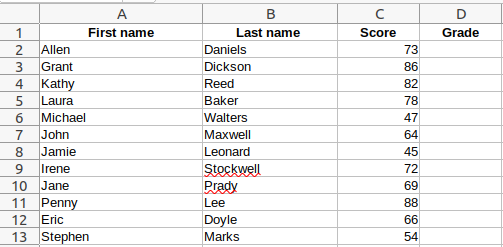The aim is to populate column D (Grade) with a more meaningful, textual grading.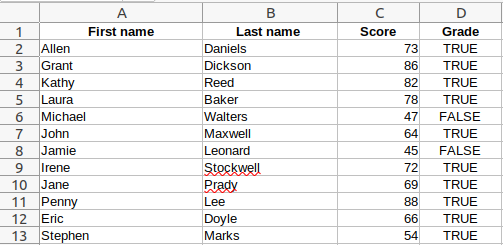=IF(C2>=50)

In its simplest form, the IF function takes a single expression.

The expression evaluates to either TRUE or FALSE, which is also what is printed by default.

The expression =IF(C2>=50) simply tests to see if the exam score is equal to 50 or above.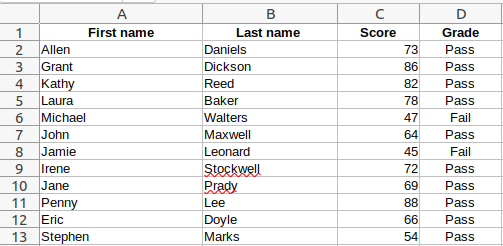=IF(C2>=50, “Pass”, “Fail”)

However, printing TRUE or FALSE is not very meaningful. Printing Pass or Fail on the other hand, would be far more appropriate.

Apart from the expression C2>=50, which is required as the first parameter, the IF function also accepts a second parameter, that executes if the expression evaluates to TRUE, and a third parameter if it evaluates to FALSE.

In our example, if the exam score is above or equal to 50, the IF function will print “Pass”, otherwise it will print “Fail”.

This is essentially equivalent to the basic IF ELSE construct.

```IF (Score >= 50) OUTPUT “Pass” ELSE OUTPUT “Fail”```

Oftentimes though, there are more than just two options to choose between.

What if the grading assigned to each student needed to be more specific, such as Pass, Credit, and Honors, where Honors signifies the best possible grading.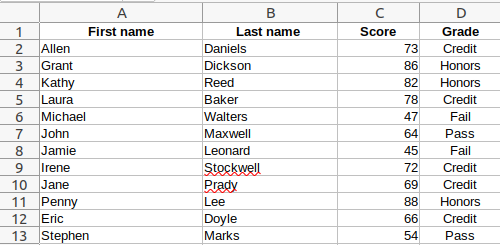=IF(C2>=80,”Honors”, IF(C2>=65,”Credit”, IF(C2>=50,”Pass”,”Fail”)))

The above formula accounts for the four possible grades (Honors, Credit, Pass, and Fail), by nesting IF function calls.

In essence, it is imitating the IF ELSE IF construct.

```IF (Score >= 80) OUTPUT “Honors” ELSE IF (Score >= 65) OUTPUT “Credit” ELSE IF (Score >= 50) OUTPUT “Pass” ELSE OUTPUT “Fail”```

While it may not be immediately obvious, the preceding formula reveals another feature of the IF function.

Apart from outputting text, the second and third parameters can also hold other expressions, or calls to other functions.

While the above formula works well, it is hard to read and makes keeping track of open and closed parentheses difficult, especially as more nested IFs are added. Fortunately, Excel provides the IFS function that replaces multiple nested IF statements, and is much easier to read.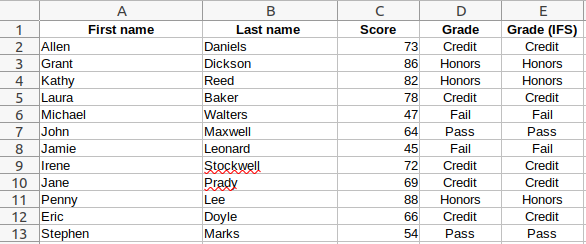The new formula using the function IFS has been added to column E. It produces the same results as the original IF formula.

The previous formula,

=IF(C2>=80,”Honors”, IF(C2>=65,”Credit”, IF(C2>=50,”Pass”,”Fail”)))

now becomes the much simpler (to read)

=IFS(C2>=80, “Honors”, C2>=65, “Credit”, C2>=50, “Pass”, TRUE, “Fail”)

The last condition in the IFS function call, TRUE, “Fail”, is a catch all statement for when all the other conditions fail.

It merely ensures that if none of the conditions match, something meaningful is still output.

Before closing off, the conditional expressions in the IF and IFS function calls used in this article have all been numeric, but text expressions such as C2=”Friday” (i.e. do the contents of cell C2 equal the text Friday), could just as easily have been used.

The only requirement is that the expression must evaluate to TRUE or FALSE.

We hope this tutorial has shown you how to run Multiple IF Statements in Microsoft Excel with examples.

Costas has a wide range of experience in Information Technology covering computer hardware, programming, telecommunications, networking, web services, and general IT support. He's worked in various roles such as PHP programmer and web developer, technical and desktop support, hardware repair, system administration. Costas has excellent background in Microsoft Windows and Office Suite (Excel, Word, Powerpoint, etc), as well as a thorough understanding of Networking and Hardware maintenance.